# chapter5_Phys201_Summer07.pdf

27 de May de 2023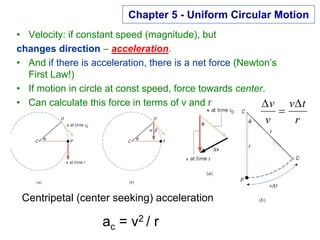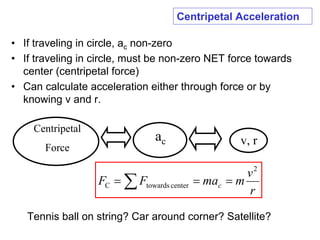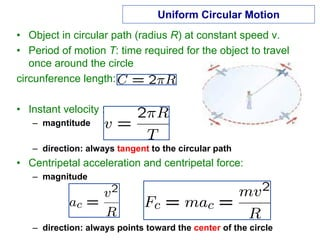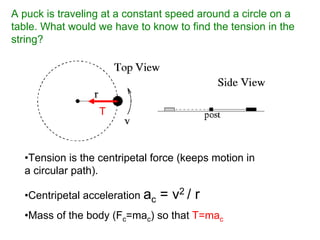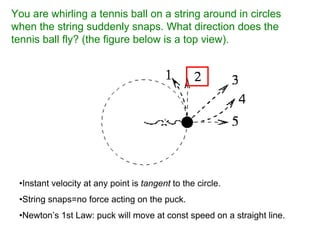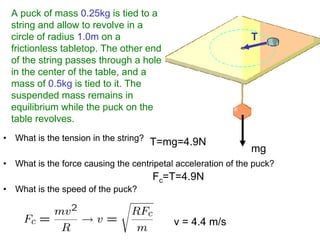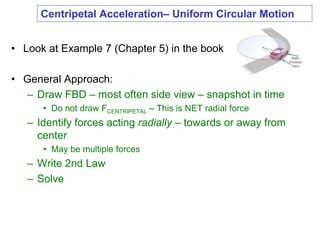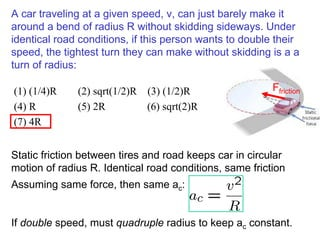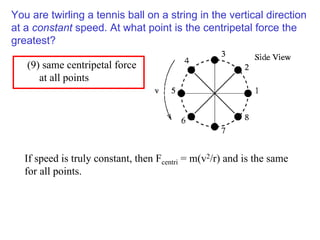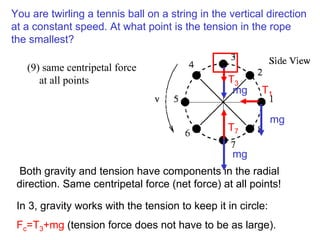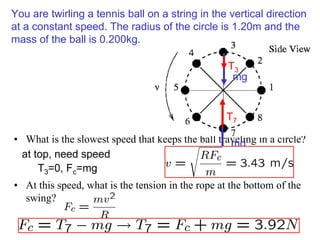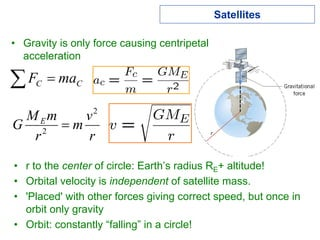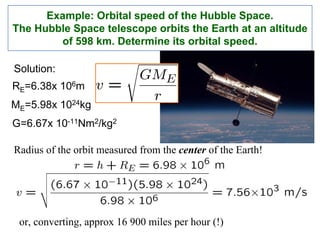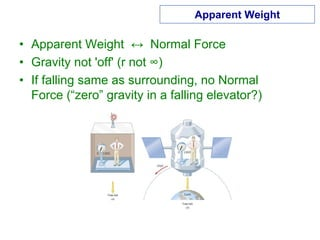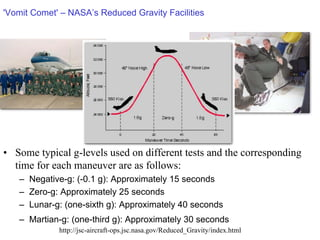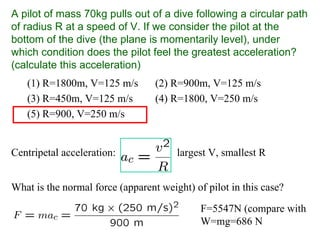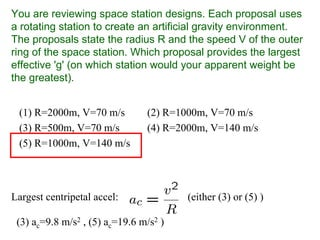1 de 17

### chapter5_Phys201_Summer07.pdf

• 1. Chapter 5 - Uniform Circular Motion • Velocity: if constant speed (magnitude), but changes direction – acceleration. • And if there is acceleration, there is a net force (Newton’s First Law!) • If motion in circle at const speed, force towards center. • Can calculate this force in terms of v and r r t v v v ∆ = ∆ Centripetal (center seeking) acceleration ac = v2 / r
• 2. Centripetal Acceleration • If traveling in circle, ac non-zero • If traveling in circle, must be non-zero NET force towards center (centripetal force) • Can calculate acceleration either through force or by knowing v and r. Centripetal Force ac v, r Tennis ball on string? Car around corner? Satellite? ∑ = = = r v m ma F F c 2 center towards C
• 3. Uniform Circular Motion • Object in circular path (radius R) at constant speed v. • Period of motion T: time required for the object to travel once around the circle circunference length: • Instant velocity : – magntitude – direction: always tangent to the circular path • Centripetal acceleration and centripetal force: – magnitude – direction: always points toward the center of the circle
• 4. A puck is traveling at a constant speed around a circle on a table. What would we have to know to find the tension in the string? •Tension is the centripetal force (keeps motion in a circular path). •Centripetal acceleration ac = v2 / r •Mass of the body (Fc=mac) so that T=mac T
• 5. You are whirling a tennis ball on a string around in circles when the string suddenly snaps. What direction does the tennis ball fly? (the figure below is a top view). •Instant velocity at any point is tangent to the circle. •String snaps=no force acting on the puck. •Newton’s 1st Law: puck will move at const speed on a straight line.
• 6. A puck of mass 0.25kg is tied to a string and allow to revolve in a circle of radius 1.0m on a frictionless tabletop. The other end of the string passes through a hole in the center of the table, and a mass of 0.5kg is tied to it. The suspended mass remains in equilibrium while the puck on the table revolves. • What is the tension in the string? • What is the force causing the centripetal acceleration of the puck? • What is the speed of the puck? T=mg=4.9N Fc=T=4.9N v = 4.4 m/s mg T
• 7. • Look at Example 7 (Chapter 5) in the book • General Approach: – Draw FBD – most often side view – snapshot in time • Do not draw FCENTRIPETAL – This is NET radial force – Identify forces acting radially – towards or away from center • May be multiple forces – Write 2nd Law – Solve Centripetal Acceleration– Uniform Circular Motion
• 8. A car traveling at a given speed, v, can just barely make it around a bend of radius R without skidding sideways. Under identical road conditions, if this person wants to double their speed, the tightest turn they can make without skidding is a a turn of radius: (1) (1/4)R (2) sqrt(1/2)R (3) (1/2)R (4) R (5) 2R (6) sqrt(2)R (7) 4R Assuming same force, then same ac: If double speed, must quadruple radius to keep ac constant. Ffriction Static friction between tires and road keeps car in circular motion of radius R. Identical road conditions, same friction
• 9. You are twirling a tennis ball on a string in the vertical direction at a constant speed. At what point is the centripetal force the greatest? (9) same centripetal force at all points If speed is truly constant, then Fcentri = m(v2/r) and is the same for all points.
• 10. You are twirling a tennis ball on a string in the vertical direction at a constant speed. At what point is the tension in the rope the smallest? (9) same centripetal force at all points Both gravity and tension have components in the radial direction. Same centripetal force (net force) at all points! mg T3 mg T7 mg T1 In 3, gravity works with the tension to keep it in circle: Fc=T3+mg (tension force does not have to be as large).
• 11. You are twirling a tennis ball on a string in the vertical direction at a constant speed. The radius of the circle is 1.20m and the mass of the ball is 0.200kg. • What is the slowest speed that keeps the ball traveling in a circle? • At this speed, what is the tension in the rope at the bottom of the swing? mg T7 mg T3 at top, need speed T3=0, Fc=mg
• 12. Satellites • Gravity is only force causing centripetal acceleration ∑ = C C ma F r v m r m M G E 2 2 = • r to the center of circle: Earth’s radius RE+ altitude! • Orbital velocity is independent of satellite mass. • 'Placed' with other forces giving correct speed, but once in orbit only gravity • Orbit: constantly “falling” in a circle!
• 13. Example: Orbital speed of the Hubble Space. The Hubble Space telescope orbits the Earth at an altitude of 598 km. Determine its orbital speed. Radius of the orbit measured from the center of the Earth! Solution: RE=6.38x 106m ME=5.98x 1024kg G=6.67x 10-11Nm2/kg2 or, converting, approx 16 900 miles per hour (!)
• 14. Apparent Weight • Apparent Weight ↔ Normal Force • Gravity not 'off' (r not ∞) • If falling same as surrounding, no Normal Force (“zero” gravity in a falling elevator?)
• 15. 'Vomit Comet' – NASA’s Reduced Gravity Facilities • Some typical g-levels used on different tests and the corresponding time for each maneuver are as follows: – Negative-g: (-0.1 g): Approximately 15 seconds – Zero-g: Approximately 25 seconds – Lunar-g: (one-sixth g): Approximately 40 seconds – Martian-g: (one-third g): Approximately 30 seconds http://jsc-aircraft-ops.jsc.nasa.gov/Reduced_Gravity/index.html
• 16. A pilot of mass 70kg pulls out of a dive following a circular path of radius R at a speed of V. If we consider the pilot at the bottom of the dive (the plane is momentarily level), under which condition does the pilot feel the greatest acceleration? (calculate this acceleration) (1) R=1800m, V=125 m/s (2) R=900m, V=125 m/s (3) R=450m, V=125 m/s (4) R=1800, V=250 m/s (5) R=900, V=250 m/s What is the normal force (apparent weight) of pilot in this case? F=5547N (compare with W=mg=686 N Centripetal acceleration: largest V, smallest R
• 17. You are reviewing space station designs. Each proposal uses a rotating station to create an artificial gravity environment. The proposals state the radius R and the speed V of the outer ring of the space station. Which proposal provides the largest effective 'g' (on which station would your apparent weight be the greatest). (1) R=2000m, V=70 m/s (2) R=1000m, V=70 m/s (3) R=500m, V=70 m/s (4) R=2000m, V=140 m/s (5) R=1000m, V=140 m/s Largest centripetal accel: (either (3) or (5) ) (3) ac=9.8 m/s2 , (5) ac=19.6 m/s2 )